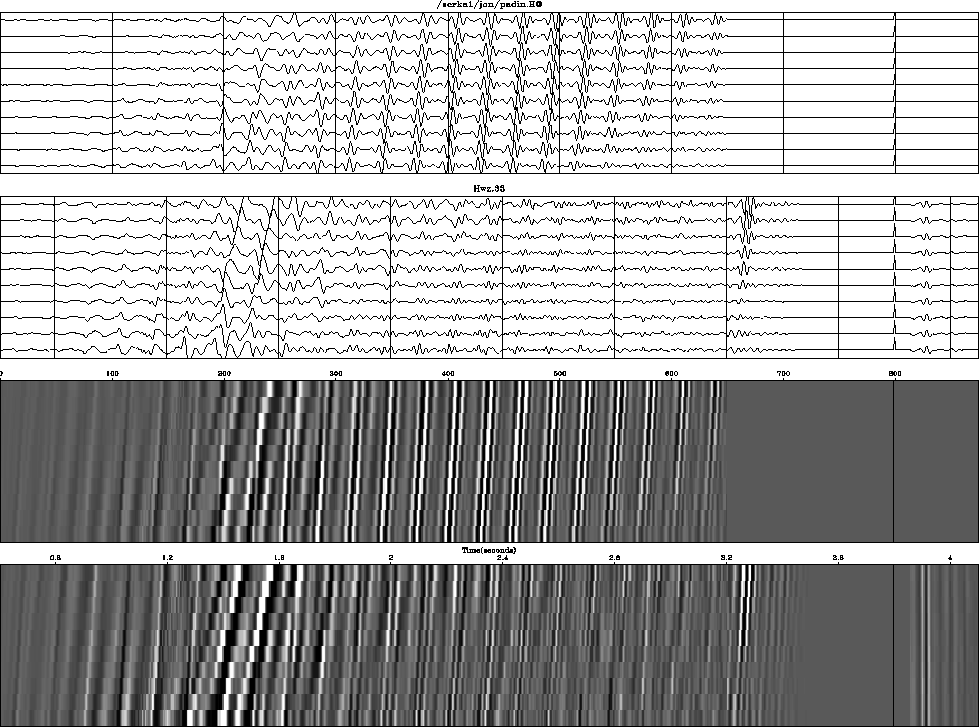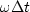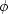Next: INTERPOLATION ERROR Up: CALCULATING ERROR FILTERS Previous: CALCULATING ERROR FILTERS

## Stabilizing technique

Theory for stabilizing least squares, using equations () and (), was described earlier in this book. I installed this stabilization, along with the filter determinations discussed in this chapter, but as I expected, stabilization in this highly overdetermined application showed no advantages. Nevertheless, it is worth seeing how stabilization is implemented, particularly since the changes to the program calling iner() make for more informative plots.

The input data is modified by appending a zero-padded impulse at the data's end. The output will contain the filter impulse response in that region. The spike size is chosen to be compatible with the data size, for the convenience of the plotting programs. The weighting function in the appended region is scaled according to how much stabilization is desired. Figure 12 shows the complete input and residual.wz33
Figure 12
Data from the North Sea (extracted from Yilmaz and Cumro dataset 33) processed by prediction error. Rightmost box is weighted according to the desired stabilization. The truncation event is weighted by zero.

It also illustrates the problem that output data flows beyond the length of the input data because of the nonzero length of the filter. This extra output is undoubtedly affected by the truncation of the data, and its energy should not be part of the energy minimization. Therefore it is weighted by zero.

## EXERCISES:

1. Given a sinusoidal function,a three-term recurrence relationship predicts xt from the previous two points, namely, xt = a1 xt-1 + a2 xt-2. Find a1 and a2 in terms of.HINT: See chapter. (Notice that the coefficients depend onbut not.)
2. Figure 9 has a separate filter for each trace. Consider the problem of finding a single filter for all the traces. What is the basic operator and its adjoint? Assemble these operators using subroutine contrunc().
3. Examine the filters on Figure 12. Notice that, besides the pulse at the water depth, another weak pulse occurs at double that depth. Suggest a physical mechanism. Suggest a mechanism relating to computational approximations.Next: INTERPOLATION ERROR Up: CALCULATING ERROR FILTERS Previous: CALCULATING ERROR FILTERS
Stanford Exploration Project
10/21/1998